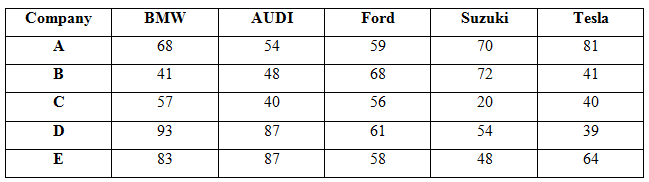# SBI Clerk Prelims 2021 Quantitative Aptitude Questions (Day-20)

Dear Aspirants, Our IBPS Guide team is providing new series of Quantitative Aptitude Questions for SBI Clerk Prelims 2020 so the aspirants can practice it on a daily basis. These questions are framed by our skilled experts after understanding your needs thoroughly. Aspirants can practice these new series questions daily to familiarize with the exact exam pattern and make your preparation effective.

Start Quiz

Approximation

Directions (01-05): What approximate value should come in the place of (?) in the following questions?

1) 20.891 * 3.981 + 45.01 % of 159.91 – √1025 * 4.96 =?

A.0

B.-1

C.-8

D.-4

E.-11

2) 54.902 % of 199.99 + 85.01 % of 139.897 +? = 160.03 % of 239.91

A.165

B.135

C.155

D.145

E.125

3) √2026 * 8.02 + 60.09 % of 149.92 +? = 19.93 * √626

A.30

B.50

C.60

D.70

E.40

4) 85.715% of 48.91 + 77.77% of 161.89 + 45.45 % of 65.99 =?

A.185

B.193

C.198

D.204

E.178

5) 66.08 * √80 – 15.97 * 29.912 + √290 * 3.19 =?

A.165

B.145

C.155

D.175

E.185

Table chart

Directions (06-10): Study the following information carefully and answer the questions given below.

The given table shows the number of five different brand cars sold in five different companies – A, B, C, D and E.6) What is the difference between the average number of BMW cars sold from E and C together and the average number of Suzuki cars sold from A and B together?

A.1

B.8

C.7

D.5

E.None of these

7) What is the ratio of the total number cars sold from D in all the brands together to the total number of cars sold from B in all the brands together?

A.167: 135

B.165: 131

C.169: 134

D.140: 111

E.None of these

8) What is the average number of Tesla cars sold in all the companies together?

A.45

B.55

C.65

D.75

E.None of these

9) The number of AUDI cars sold from E is approximately what percent of the total number of cars sold from A in all the brands together?

A.26%

B.28%

C.30%

D.32%

E.20%

10) What is the difference between the total number of AUDI and Ford brand cars sold from all the companies together?

A.15

B.16

C.17

D.18

E.None of these

20.891 * 3.981 + 45.01 % of 159.91 – √1025 * 4.96 =?

21 * 4 + 45/100 * 160 – √1024 * 5 =?

84 + 72 – 160 =?

-4 =?

54.902 % of 199.99 + 85.01 % of 139.897 +? = 160.03 % of 239.91

55/100 * 200 + 85/100 * 140 +? = 160/100 * 240

110 + 119 +? = 384

? = 155

√2026 * 8.02 + 60.09 % of 149.92 +? = 19.93 * √626

√2025 * 8 + 60/100 * 150 +? = 20 * √625

360 + 90 +? = 500

? = 50

85.715% of 48.91 + 77.77% of 161.89 + 45.45 % of 65.99 =?

6/7 * 49 + 7/9 * 162 + 5/11 * 66 =?

42 + 126 + 30 =?

198 =?

66.08 * √80 – 15.97 * 29.912 + √290 * 3.19 =?

66 * √81 – 16 * 30 + √289 * 3 =?

594 – 480 + 51 =?

165 =?

Average of BMW cars sold from C and E = (57 + 83)/2 = 70

Average of Suzuki employee from A and B = (70 + 72)/2= 71

Difference = 71 – 70 = 1

Required ratio = (93 + 87 + 61 + 54 + 39): (41 + 48 + 68 + 72 + 41)

= 334: 270

= 167: 135

Average = (81 + 41 + 40 + 39 + 64)/5

= 53

Required ratio = 87/(68 + 54 + 59 + 70 + 81) * 100

= 26%

AUDI = 54 + 48 + 40 + 87 + 87 = 316

Ford = 59 + 68 + 56 + 61 + 58 = 302

Difference = 316 – 302 = 14

 Check Here to View SBI Clerk Prelims 2021 Quantitative Aptitude Questions Day – 19 Day – 18 Day – 17
5 1 vote
Rating